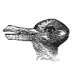# Visualizing Clusters

Consider the following dataset, with (only) ten points

```x=c(.4,.55,.65,.9,.1,.35,.5,.15,.2,.85)
y=c(.85,.95,.8,.87,.5,.55,.5,.2,.1,.3)
plot(x,y,pch=19,cex=2)```We want to get – say – two clusters. Or more specifically, two sets of observations, each of them sharing some similarities.

Since the number of observations is rather small, it is actually possible to get an exhaustive list of all partitions, and to minimize some criteria, such as the within variance. Given a vector with clusters, we compute the within variance using

```within_var = function(I){
I0=which(I==0)
I1=which(I==1)
xbar0=mean(x[I0])
xbar1=mean(x[I1])
ybar0=mean(y[I0])
ybar1=mean(y[I1])
w=sum(I0)*sum( (x[I0]-xbar0)^2+(y[I0]-ybar0)^2 )+
sum(I1)*sum( (x[I1]-xbar1)^2+(y[I1]-ybar1)^2 )
return(c(I,w))
}```

Then, to compute all possible partitions, use

```base2=function(z,n=10){
Base.b=rep(0,n)
ndigits=(floor(logb(z, base=2))+1)
for(i in 1:ndigits){
Base.b[ n-i+1]=(z%%2)
z=(z%/%2)}
return(Base.b)}
L=function(x) within_var(base2(x))
S=sapply(1:(2^10),L)```

The cluster indices at the mimimum is here

```I=S[1:n,which.min(S[n+1,])]
```

To visualize those clusters, use

```cluster_viz = function(indices){
library(RColorBrewer)
CL2palette=rev(brewer.pal(n = 9, name = "RdYlBu"))
CL2f=CL2palette[c(1,9)]
plot(x,y,pch=19,xlab="",ylab="",xlim=0:1,ylim=0:1,cex=2,col=CL2f[1+I])
CL2c=CL2palette[c(3,7)]
I0=which(indices==0)
I1=which(indices==1)
xbar0=mean(x[I0])
xbar1=mean(x[I1])
ybar0=mean(y[I0])
ybar1=mean(y[I1])
segments(x[I0],y[I0],xbar0,ybar0,col=CL2c)
segments(x[I1],y[I1],xbar1,ybar1,col=CL2c)
points(xbar0,ybar0,pch=19,cex=1.5,col=CL2c)
points(xbar1,ybar1,pch=19,cex=1.5,col=CL2c)}```

and then, simply

`cluster_viz(I)`But that was possible only because is not to large (since the total number of scenarios – with only 2 clusters – is , or if we changes zeros in ones).

One idea can be to use hierarchical clustering techniques. For instance, with a complete aggregation technique, we get

```H=hclust(dist(cbind(x,y)),method="complete")
I=cutree(H,k=2)-1
cluster_viz(I)```If we change the aggregation technique, to Ward, we get

```H=hclust(dist(cbind(x,y)),method="ward.D")
I=cutree(H,k=2)-1
cluster_viz(I)```or, for the single one,

```H=hclust(dist(cbind(x,y)),method="single")
I=cutree(H,k=2)-1
cluster_viz(I)```Another idea is to use some -means algorithm (e.g. Lloyd’s)

```km=kmeans(cbind(x,y), centers=2, nstart = 1, algorithm = "Lloyd")
I=km\$cluster-1
cluster_viz(I)```But if we run it another time, we might have different starting points, and different clusters

```km=kmeans(cbind(x,y), centers=2, nstart = 1, algorithm = "Lloyd")
I=km\$cluster-1
cluster_viz(I)```orevenA natural strategy is to run it many times, with different starting points, and to keep the one with the smallest within-variance.

But to be honest, I like the idea that drawing different starting point will yield different clusters. Actually, we can search some kind of robustness. For instance, which observations are more likely to belong to the same cluster as the second one (on top) ?

```ref=2
M=NULL
for(s in 1:10000) {
km=kmeans(cbind(x,y), centers=2, nstart = 1, algorithm = "Lloyd")
I=km\$cluster-1
I=(I[ref]==1)*I+(I[ref]==0)*(1-I)
M=rbind(M,I) }
M_prop=apply(M,2,mean)```or the fifth one (on the left)## 12 thoughts on “Visualizing Clusters”

1.Panos says:

Nice work! Thanks for sharing.

I think CL2cl=CL2palette[c(3,7)] should change to:
CL2c=CL2palette[c(3,7)]

2.Robert Hagan says:

Thanks.. the output is very useful. Hope you can soon post the corrected code.. Thanks for posting an insight.

3.Janet says:

Very useful post. Thank you! Could you post your code for the last two charts to color the points and label with M_prop?

Also, the palette variable is inconsistently named CL2cl and CL2c. Either `CL2cl=CL2palette[c(3,7)]` should change to `CL2c=CL2palette[c(3,7)]` or the last three lines should reference CL2cl instead of CL2c.

1.Arthur Charpentier says:

indeed… I changed the name when uploading the post ! I will fix it !

4.Charles Cara says:

Very useful post. Thank you

5.Fr. says:

Since the n argument is trapped in a function call, you need to modify the line that finds the cluster indices at their minimum for the code to run straight out of the post. This should work:

I = S[ 1:(nrow(S) – 1), which.min(S[ nrow(S), ])]

1.Arthur Charpentier says:

indeed, sorry
I made a quick copy and paste with some small changes.. as always, there are some typos…
thanks François !

1.François says:

No problem at all.

I discovered the bug because I wanted to rewrite your code with ggplot2 and any number of clusters. Not sure how to do the last bit, though.

This site uses Akismet to reduce spam. Learn how your comment data is processed.